• map<T1, T2> m; m.insert(pair<T1, T2>(key, value)); #include <iostream> #include <map> using namespace std; int main() { map<string, int> m; m.insert(pair<string, ...

create

1. insert

map<T1, T2> m;
m.insert(pair<T1, T2>(key, value));

#include <iostream>
#include <map>
using namespace std;
int main() {
map<string, int> m;
m.insert(pair<string, int>("1", 000));
m.insert(make_pair<string, int>("2", 111));
m.insert(pair<string, int>("2", 222));
cout << m["1"] << endl;
cout << m["2"] << endl;
return 0;
}

0
111

insert will not cover the previous key-value.

2. [key] = value

map<T1, T2> m;
m[key] = value;

example

#include <iostream>
#include <map>
using namespace std;
int main() {
map<string, int> m;
m["1"] = 000;
m["2"] = 111;
m["2"] = 222;
cout << m["1"] << endl;
cout << m["2"] << endl;
return 0;
}

0
222

3.init

#include <iostream>
#include <unordered_map>
using namespace std;
int main() {

unordered_map<char, char> pair = {
{')', '('},
{']', '['},
{'}', '{'},
};

cout << pair[')'];

return 0;
}

(

this will cover previous key-value

retrieve (key)

1. count

map<T1, T2> m;
if(m.count(key) > 0)
{
return m[key];
}
return null;

example

#include <iostream>
#include <map>
using namespace std;
int main() {
map<string, int> m;
int result;
if (m.count("1") > 0) result = m["1"];
else result = -1;
cout << result;
return 0;
}

-1

if the key in map , it will traverse twice, m.count() once, m[“1”] once,
it is inefficient compared next method.

2. find

map<T1, T2> m;
map<T1, T2>::iterator it = m.find(key);
if(it != m.end())
{
return m[key];
}
return null;

example

#include <iostream>
#include <map>
using namespace std;
int main() {
map<string, int> m;
int result;
auto it = m.find("1");
if (it != m.end()) result = it->second;
else result = -1;
cout << result << endl;

m["1"] = 111;
it = m.find("1");
if (it != m.end()) result = it->second;
else result = -1;
cout << result << endl;
return 0;
}

-1
111

if key in map, this just need once traverse.

update

1. [key] = value

it can create and update. usage showed on create table.

delete

1. erase by key

map<T1, T2> m;
m.erase(key);

example

#include <iostream>
#include <map>
using namespace std;
int main() {
map<string, int> m;
m["1"] = 111;
m["2"] = 222;
for (auto it = m.begin(); it != m.end(); it++) cout << it->second << endl;
cout << endl;

m.erase("2");
for (auto it = m.begin(); it != m.end(); it++) cout << it->second << endl;
cout << endl;

m.erase("3");
for (auto it = m.begin(); it != m.end(); it++) cout << it->second << endl;
return 0;
}

111
222

111

111

if the key not exists, it will delete nothing

2. erase by value

map<T1, T2> m;
map<T1, T2>::iterator i;
for (auto j = m.begin(); j != m.end(); j++)
if (j->second == value)
i = j;
m.erase(i);

example

#include <iostream>
#include <map>
using namespace std;
int main() {
map<string, int> m;
m["1"] = 111;
m["2"] = 222;
for (auto & it : m) cout << it.second << endl;
cout << endl;

map<string, int>::iterator i;
for (auto j = m.begin(); j != m.end(); j++)
if (j->second == 222)
i = j;
m.erase(i);

for (auto & it : m) cout << it.second << endl;
return 0;
}

111
222

111

erase can delete the iterator pointed item

wrong example

for (auto j = m.begin(); j != m.end(); j++)
if (j->second == 222)
m.erase(j);

can’t delete while traversing, it will cause segmentation fault.

other

2. size()

example

#include <iostream>
#include <map>
using namespace std;
int main() {
map<string, int> m;
cout << m.empty() << endl;
cout << m.size() << endl;
return 0;
}

1
0

feature

1. counter

#include <iostream>
#include <map>
using namespace std;
int main() {
map<string, int> m;
cout << m["111"] << endl;

m["222"]++;
cout << m["222"] << endl;

cout << m.size() << endl;
return 0;
}

0
1
2

if value type is int, it can be a counter for the key

展开全文c++
• 一、map 内部实现机理 map内部实现了一个 红黑树（红黑树是非严格平衡二叉搜索树，而AVL是严格平衡二叉搜索树）， 红黑树具有自动排序的功能，因此map内部的所有元素都是有序的，红黑树的每一个节点都代表着map的一...

一、map

内部实现机理

map内部实现了一个 红黑树（红黑树是非严格平衡二叉搜索树，而AVL是严格平衡二叉搜索树）， 红黑树具有自动排序的功能，因此map内部的所有元素都是有序的红黑树的每一个节点都代表着map的一个元素。因此，对于map进行的查找，删除，添加等一系列的操作都相当于是对红黑树进行的操作。map中的元素是按照二叉搜索树（又名二叉查找树、二叉排序树，特点就是左子树上所有节点的键值都小于根节点的键值，右子树所有节点的键值都大于根节点的键值）存储的，使用中序遍历可将键值按照从小到大遍历出来。

优缺点以及适用处

1. 优点：有序性，这是map结构最大的优点，其元素的有序性在很多应用中都会简化很多的操作；红黑树，内部实现一个红黑树使得map的很多操作在O(logN)的时间复杂度下就可以实现，因此效率非常的高。

2. 缺点：空间占用率高，因为map内部实现了红黑树，虽然提高了运行效率（低于unorder_map），但是因为每一个节点都需要额外保存父节点、孩子节点和红/黑性质，使得每一个节点都占用大量的空间（但占用的内存比unorder_map低）

3. 适用处：对于那些数据存储有顺序要求的问题，用map会更高效一些

使用举例

vector<int> list = { 5,14,34,22,39,5 };
map<int, int> map;
for (int i = list.size() - 1; i >= 0; i--) {
map[i] = list[i];  //倒序插入
}
for (auto i = map.begin(); i != map.end(); i++) {
cout << i->first << ' ' << i->second << endl;  //输出的数是有序的且有两个5
}
if (map.find(3) != map.end()) {
cout << "find key=" << map.find(3)->first << ", value=" << map.find(3)->second << endl;
}
if (map.count(5) > 0) {  //m.count(n)计算下标为n的位置有无数据，有返回1，无返回0
cout << "count 5: " << map.count(5) << endl;  //find()和count()的输入参数都是key值
}

map是基于RBT的，因此元素是有序存储的（默认按 的升序排列）。

输出结果：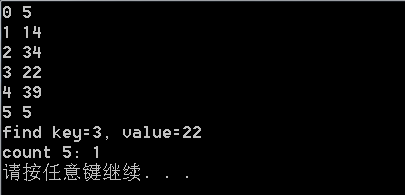二、unordered_map

内部实现机理

unordered_map内部实现了一个 哈希表（也叫散列表，通过把关键码值映射到Hash表中一个位置来访问记录，查找的时间复杂度可达到O(1)，其在海量数据处理中有着广泛应用）。因此， 其元素的排列顺序是无序的

优缺点以及适用处

1. 优点： 因为内部实现了哈希表，因此其查找速度非常的快（运行效率快于map）
2. 缺点： 哈希表的建立比较耗费时间（unorder_map占用的内存比map要高）
3. 适用处：对于查找问题，unordered_map会更加高效一些，因此遇到查找问题，常会考虑一下用unordered_map

使用举例

vector<int> list = { 5,14,34,22,39,5 };
unordered_map<int, int> map;
for (int i = list.size()-1; i>=0; i--) {
map[i] = list[i];  //倒序插入
}
cout << map << endl;
for (unordered_map<int, int>::iterator i = map.begin(); i != map.end(); i++) {
cout << i->first << ' ' << i->second << endl;  //输出的数是有序的且有两个5
}
if (map.find(3) != map.end()) {
cout << "find key=" << map.find(3)->first << ", value=" << map.find(3)->second << endl;
}
if (map.count(5) > 0) {  //m.count(n)计算下标为n的位置有无数据，有返回1，无返回0
cout << "find 5: " << map.count(5) << endl;  //find()和count()的输入都是key值
}

unordered_map 是基于hash表的，因此元素是无序存储的（ 不按键 升序排列）。

输出结果：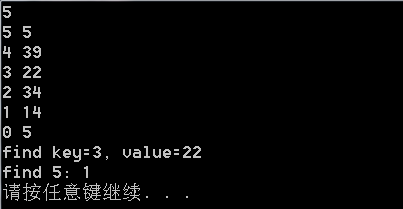三、set

内部实现机理

set实现了红黑树的平衡二叉检索树的数据结构，插入元素时，它会自动调整二叉树的排列，把元素放到适当的位置，以保证每个子树根节点键值大于左子树所有节点的键值，小于右子树所有节点的键值；另外，还得保证根节点左子树的高度与右子树高度相等。在set中每个元素的值都唯一，而且系统能根据元素的值自动进行排序。平衡二叉检索树使用中序遍历算法，检索效率高于vector、deque和list等容器，另外使用中序遍历可将键值按照从小到大遍历出来。

使用举例

vector<int> list = { 5,14,34,22,39,5 };
set<int> set1;
for (int i = list.size() - 1; i >= 0; i--) {
set1.insert(list[i]);  //倒序插入
}
for (auto i = set1.begin(); i != set1.end(); i++) {
cout << *i << endl;  //输出的数是有序的且只有一个5
}
cout << "find 5: " << *set1.find(5) << endl;
cout <<"count 5: " << set1.count(5) << endl;

set 是基于RBT的，因此元素是顺序存储的（默认按 键值 升序排列）。

输出结果：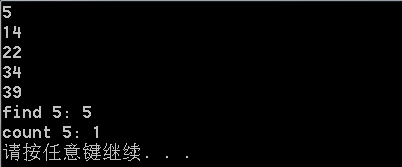四、unordered_set

内部实现机理

unordered_set的内部实现了一个 哈希表，因此， 其元素的排列顺序是无序的

使用举例

vector<int> list = { 5,14,34,22,39,5 };
unordered_set<int> set;
for (int i = list.size() - 1; i >= 0; i--) {
set.insert(list[i]);  //倒序插入
}
for (unordered_set<int>::iterator i = set.begin(); i != set.end(); i++) {
cout << *i << endl;  //输出的数是无序的且只有一个5
}
cout << "find 39: " << *set.find(39) << endl;
cout << "count 14:" << set.count(14) << endl;

unordered_set 是基于hash表的，因此元素是无序存储的（ 不按键值 升序排列）。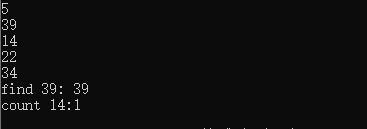五、总结

数据结构mapunordered_mapsetunordered_set
实现机理红黑树hash表红黑树hash表
元素格式key+valuekey+valuekeykey
存储规律键升序无序键升序无序
元素重复键不可,值可键不可,值可不可重复不可重复
头文件#include< map>#include<unordered_map>#include< set>#include<unordered_set>
展开全文c++ stl
• set 、map底层是红黑树，需要显性提供排序规则： set 使用lambda表达式 auto fn = [](Node x,Node y)->decltype(bool()) { return x.a+x.b>y.a+y.b; }; set<Node,decltype(fn)> s(fn); s.insert...

set 、map底层是红黑树，需要显性提供排序规则：
set 使用lambda表达式

auto fn = [](Node x,Node y)->decltype(bool())
{
return x.a+x.b>y.a+y.b;
};
set<Node,decltype(fn)> s(fn);
s.insert(Node({1,2}));

set 重载类型<

struct Node{
int a;
int b;
bool operator<(Node c) const
{
return a+b>c.a+c.b;
}
};
set<Node> s1;
s1.insert(Node({3,4}));

multiset 、map 、multimap
都需要指定排序规则
而unordered_开头的，底层哈希表，需要指定哈希函数以及重载自定义类型的==，因为hash表不排序，用hash函数做映射，用==判断是否重复

展开全文• map unordered_map

map是按照压入顺序来储存数据的；

unordered_map是不按照压入顺序来储存数据的。

实验代码如下：

#include<iostream>
#include<stack>
#include<map>
#include<unordered_map>

using namespace std;

int main(){
map<int,int> map1;//声明两种map
unordered_map<int,int> map2;
for(int i = 0 ; i < 5 ; i++ ){//都给两种map顺序输入数字0 2 4 6 8
map1[i] = i*2;
map2[i] = i*2;
}
for(auto it=map1.begin(); it!=map1.end(); it++){//各自按照储存顺序输出
cout<<it->second<<' ';
}
cout<<endl;
for(auto it=map2.begin(); it!=map2.end(); it++){
cout<<it->second<<' ';
}
int a;//维持窗口，别的方法实在不好用QAQ
cin>> a;
}

结果如下：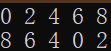展开全文c++ map
• std::map对应的数据结构是红黑树。...所以对于需要高效率查询的情况，使用std::unordered_map容器，但是std::unordered_map对于迭代器遍历效率并不高。 而如果对内存大小比较敏感或者数据存储要求有序的话，.c++
• c++中mapunordered_map的区别 头文件 map: #include < map > unordered_map: #include < unordered_map > 内部实现机理 mapmap内部实现了一个红黑树，该结构具有自动排序的功能，因此map内部的...c++
• Input: nums = [2,7,11,15], target = 9 Output: [0,1] class Solution { public int[] twoSum(int[] nums, int target) { //hashmap key存储数组值，value存数组... map = new HashMap<Integer,Integer>();hashmap java c++
• 之前写过一篇：C++98 使用无序map C++98 使用无序map vs2017 Release X64环境下： 执行一千万次的时间下图所示： 第一组数据： 可以看出有序map执行插入所需时间比较短，约快2.3倍， 第二组数据： 遍历时间基本一致...c++ map
• unordered_map 查找O(1) map 查找O(logn)c++
• class Solution { public: vector<int> singleNumber(vector<... unordered_map<int, int> freq; for (int num: nums) { ++freq[num]; } vector<int> ans; for (const auto&.c++ 容器
• mapunordered_map都是键值对的储存结构。但二者存在不同，运用场景也不同。 std::map map支持键值的自动排序，底层机制是红黑树，红黑树的查询和维护时间复杂度均为O(logn)O(logn)O(logn)，但是空间占用比较大. ...操作系统 服务器 网络
• 本篇学习unordered_map的插入数据操作，具体的函数如下： insert(C++11)插入元素或结点 (C++17 起) insert_or_assign(C++17)插入元素，或若键已存在则赋值给当前元素 emplace(C++11)原位构造元素 emplace_hint(C++11...
• 本质区别在于std::map底层使用红黑树，而std::unordered_map使用的是hash map。 1、std::map 头文件：<map> 类声明： template< class Key, class T, class Compare = std::less<Key>, ...hashmap c++11
• 1，map简介 map是STL的一个关联容器，它提供一对一的hash。 第一个可以称为关键字(key)，每个关键字只能在map中出现一次； 第二个可能称为该关键字的值(value)； map以模板(泛型)方式实现，可以存储任意类型的...stl
• map、set、unordered_mapunordered_set在C++中使用频率较高的几种数据存储结构，因此，熟练掌握其基本的方法对于深入学习C++就先得尤为重要 1、map 2、set 3、unordered_map 4、unordered_set
•  ...unordered_map底层使用的数据结构是哈希表（hash table），因此在unordered_map中查找、添加或删除元素时间复杂度都是常数时间O(1)。此外，unordered_map中的元素是无序的。哈希表 c++
• C++ mapunordered_map详解 官方文档链接 概述  C++中mapunordered_map提供的是一种键值对容器，在实际开发中会经常用到，它跟Python的字典很类似，所有的数据都是成对出现的,每一对中的第一个值称之为关键字...c++
• C++ mapunordered_map区别及使用mapunordered_map区别1. 引入的头文件不同2. 内部实现机理不同3. 优缺点以及适用处mapunordered_map使用参考 文章转自： ...c++
• 2. 容量为100的时候，查找效率：map = unordered_map > hash_map 3. 容量为1000的时候，查找效率：unordered_map > hash_map > 4倍map 4. 容量为1万的时候，查找效率：hash_map > unordered_map > ...c++
• 目录 map/multimap和unordered_map容器 map基本概念 map构造和赋值 map大小和交换 map插入和删除 map查找和统计 map容器排序 unordered_map的使用 mapunordered_map区别： 例：leetcode3.无重复字符的最长子串 ...c++ 开发语言 容器 数据结构 stl
• mapunordered_map的遍历方法是相同的，不过遍历结果，map是有序的，unoredred_map遍历是无序的。 std:map 是个有序的关系容器，其完整原型如下： template< class Key, class T, class Compare=std::less<...map
• c++ unordered_map4种遍历方式

千次阅读 2021-12-17 19:25:20
c++ unordered_map4种遍历方式方式一：值传递遍历方式二：引用传递遍历方式三：使用迭代器遍历方式四：结构化绑定(c++17特性) 首先定义个unordered_map unordered_map<int,int> map={ pair<int,int>(1,...c++ 开发语言 map
• unordered_set和unordered_map用法详解

千次阅读 多人点赞 2021-07-17 00:02:28
文章目录1.unordered_map1.介绍2.性质3.模板4.定义迭代器5.功能函数5.1构造函数5.2 容量操作：size、empty5.3 元素操作：find、insert、at、erase、clear、swap、for循环打印5.4 迭代器和bucket操作2.unordered_set...stl
• mapunordered_map使用分别需要下面这两个头文件： #include<map> #include<unordered_map> map内部实现了一个红黑树（非严格平衡二叉搜索树），红黑树可以自动排序，因此map内部所有元素都是有序的，...
• C++语言mapunordered_map的下标操作 C++语言mapunordered_map的下标操作
• C++STL中，mapunordered_map插入数据效率对比概述运行环境测试代码运行结果测试结果参考网址 概述 对于一般印象而言，有序的map容器比无序的unordered_map容器的插入效率要低一点，本次测试比较两种容器使用insert...c++ stl
• c[k] 返回关键字为k的元素: 如果k不在c中，添加一个关键字为k的元素，对其进行值初始化 c.at(k) 访问关键字为k的元素，带参数检查；若k不在c中，抛出一个out_of_range异常
• C++_STL——unordered_mapunordered_multimap、unordered_set、unordered_multiset 参考：cplusplus 这几种都是拉链法所写的，所以放在一起他们有很多相似之处，以下只记录之前没遇到过的成员函数 遇到不清楚...c++
•数据结构 c++ map
• hash_mapunordered_mapmap的效率、区别和分析 一、前言 二、三者的实现区别 map hash_mapunordered_map 三、三者查询效率高低 时间效率 三者使用选择 例题： 编译器报错解决方法 一、......